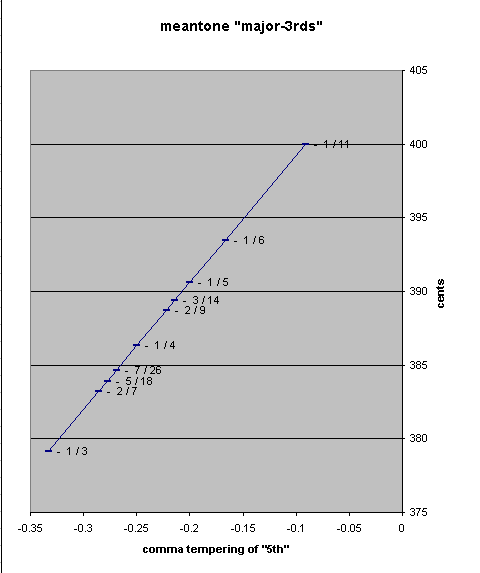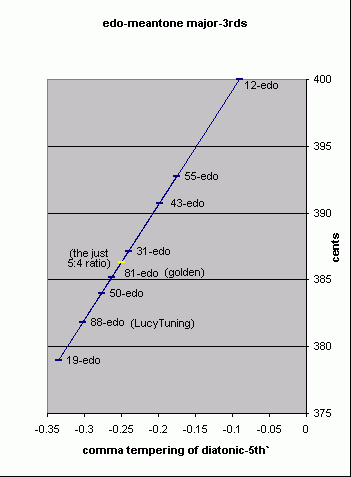# major-3rd / major third / maj3 / M3

[Joe Monzo]

A diatonic interval composed of 2 tones (i.e., "whole-tones"), whose diatonic pitch distance always traverses 3 letter-names inclusively (which may or may not involve a change of accidental), and which is approximately 400 cents (give or take about 50). For example, in the key of C-major ("t" = tone, "s" = diatonic-semitone) :

```   M2        M2    m2     M2       M2       M2     m2
t         t     s      t        t        t      s
C        D        E    F        G        A        B    C   =  C-major diatonic scale
|                 |
--- major-3rd----

major-3rd = 2t + 0s
= 2(M2)
```

Some examples of major-3rds which include accidentals:

• Abb : Cb
• Ab : C
• A : C#
• A# : Cx
• etc.

The standard 12edo major-3rd is the one of 400 cents. Some typical JI or rational values are given below in John Chalmers's definition.

Successively closer small-integer rational approximations of the 12-edo major-3rd are:

```ratio    ~cents

14/11  417.5079641
5/4   386.3137139
19/15  409.2443014 (good approximation of Pythagorean 3rd)
24/19  404.4419847
29/23  401.3028469
34/27  399.0904069
63/50  400.1084805 (only ~1/9 cent wider than 2(4/12))
```

Other 'major 3rds' can be defined mathematically by irrational numbers, generally in the context of some form of temperament.

Examples of 'major 3rds' in some common meantone tunings:

```meantone           adjustment of 81/64
"flavor"    --- [pythagorean 'major 3rd'] ---     ----- 2,3,5-monzo -----      ~ cents

1/11-comma   ((3/2)4) / (22) / ((81/80)(4*1/11)) =  [-50/11  28/11,   4/11 >   =  399.9995345
1/6-comma    ((3/2)4) / (22) / ((81/80)(4*1/6))  =  [-10/3    4/3 ,   2/3  >   =  393.4824771
1/5-comma    ((3/2)4) / (22) / ((81/80)(4*1/5))  =  [-14/5    4/5 ,   4/5  >   =  390.6149718
3/14-comma   ((3/2)4) / (22) / ((81/80)(4*3/14)) =  [-18/7    4/7 ,   6/7  >   =  389.386041
2/9-comma    ((3/2)4) / (22) / ((81/80)(4*2/9))  =  [-22/9    4/9 ,   8/9  >   =  388.7033016
1/4-comma    ((3/2)4) / (22) / ((81/80)(4*1/4))  =  [ -2      0   ,   1    >   =  386.3137139
7/26-comma   ((3/2)4) / (22) / ((81/80)(4*7/26)) =  [-22/13  -4/13,  14/13 >   =  384.6593839
5/18-comma   ((3/2)4) / (22) / ((81/80)(4*5/18)) =  [-14/9   -4/9 ,  10/9  >   =  383.9241261
2/7-comma    ((3/2)4) / (22) / ((81/80)(4*2/7))  =  [-10/7   -4/7 ,   8/7  >   =  383.2413868
1/3-comma    ((3/2)4) / (22) / ((81/80)(4*1/3))  =  [  2/3   -4/3 ,   4/3  >   =  379.1449507
```Note the frequent occurence of '4' in the numerators of the rational exponents of the monzos: this is a result of the place of the Pythagorean 'major 3rd' as the fourth member in the chain of 5ths, and the implied pythagorean ratio (2-6*)34 for the fourth member in the meantone chain.

Also note that 1/4-comma meantone gives a major-3rd with the exact JI 5:4 ratio, which is made obvious by the fact that all of the exponents in its monzo are integers.

The golden meantone "major-3rd" has the ratio 2[ (10 - 4Φ) / 11 ], where Φ = (1 + 51/2) / 2, and is ~384.8578958 cents.

Examples of 'major 3rds' in some common equal temperaments:

 EDO '3rd' cents 12 24/12 400 13 24/13 ~369 15 25/15 400 16 25/16 375 17 26/17 ~424 (note that 17-EDO has nothing that resembles the JI major-3rd of 5/4 ratio; also note the neutral-3rd of 2(5/17) = ~353 cents) 19 26/19 ~379 22 27/22 ~382 24 28/24 400 (also note the 'neutral 3rd' of 2(7/24) = 350 cents) 31 210/31 ~387 41 213/41 ~380 (also note the pseudo-Pythagorean '3rd' of 2(14/41) = ~410 cents) 43 214/43 ~391 50 216/50 384 (also note the pseudo-Pythagorean '3rd' of 2(17/50) = 408 cents) 53 217/53 ~385 (also note the pseudo-Pythagorean '3rd' of 2(18/53) = ~407.5 cents) 55 218/55 ~393 72 223/72 383 1/3

(Note that the cents values of some ET pitches are exact.)

Below is a pitch-height graph of the size of the major-3rd in various EDO-meantone tunings:. . . . . . . . .
[John Chalmers, Divisions of the Tetrachord]

An interval approximating 400 cents. Major thirds include the 5/4 (386¢) of Just Intonation, the Pythagorean Ditone of 81/64 (408¢), and more complex intervals such as 19/15 (409¢) and the Skhismic Diminished Fourth, 8192/6561 (384¢) of extended Pythagorean tuning.

. . . . . . . . .

The tonalsoft.com website is almost entirely the work of one person: me, Joe Monzo. Please reward me for my knowledge and effort by choosing your preferred level of financial support. Thank you.

 support level donor: \$5 USD friend: \$25 USD patron: \$50 USD savior: \$100 USD angel of tuning: \$500 USD microtonal god: \$1000 USD